# Worksheet on Pie Chart | Pie Chart Worksheets with Answers | Circle Graph Questions

In this Worksheet on Pie Chart, you can see sample questions and answers on the pie chart, how to solve them. The term pie means a circle and chart means the representation of data. Here, we will represent tabular data in a circle. Pie Chart contains sectors, the percentage of sector shows the value of the component.

We have also included the formula to find the central angle of each component of a pie chart on this page. Get the simple steps to convert the data into a pie chart from the following sections. To see the central angle of each component, divide the component value by the total number of components. Multiply the result with 360 degrees.

1. Rakesh yearly salary is \$ 52007. He plans his budget for a year as mentioned here:

Item Food Education Rent Savings Miscellaneous
Amount (in dollars) 12089 4468 9150 13300 13,000

Draw a pie chart for the Rakesh yearly budget.

Solution:

Given data is the Rakesh yearly budget.

Yearly Salary = \$ 52007

Central angle for a component = (Value of the component / Sum of the values of all components) * 360°

Calculation of central angles:

Item Amount (in dollars) Central Angle
Food 12089 (12089 / 52007) * 360 = 83.68°
Education 4468 (4468 / 52007) * 360 = 30.92°
Rent 9150 (9150 / 52007) * 360 = 63.33°
Savings 13300 (13300 / 52007) * 360 = 92°
Miscellaneous 13000 (13000 / 52007) * 360 = 89.98°

Steps of constructing a pie chart:

1. Draw a circle of any convenient radius.

2. Draw a horizontal radius of the circle.

3. Draw sectors starting from the horizontal radius with central angles of 83.68°, 30.92°, 63.33°, 92°, and 89.98° respectively.

4. Shade the sectors differently using different colors and label them.Thus, we obtain the required pie chart, shown in the above figure.

2. In a company there are 19200 workers of different religions. The data of the different religion are given below:

Religion Hindu Nepali Islam Christian Buddhism
No of Workers 9500 700 4000 4500 500

Draw a pie chart for the above data.

Solution:

Total Number of workers in the company = 19200

Central angle for a component = (Value of the component / Sum of the values of all components) * 360°

Calculation of central angles:

Religion No of Workers Central Angle
Hindu 9500 (9500 / 19200) * 360 = 178.12°
Nepali 700 (700 / 19200) * 360 = 13.125°
Islam 4000 (4000 / 19200) * 360 = 75°
Christian 4500 (4500 / 19200) * 360 = 84.375°
Buddhism 500 (500 / 19200) * 360 = 9.375°

Steps for constructing a pie chart

• Draw a circle of any radius.
• Draw a horizontal radius of the circle.
• draw sectors starting from the horizontal radius with the central angles of 9.37°, 84.37°, 75°, 13.12°, and 178.12°.
• Shade the sectors using different colors and label them.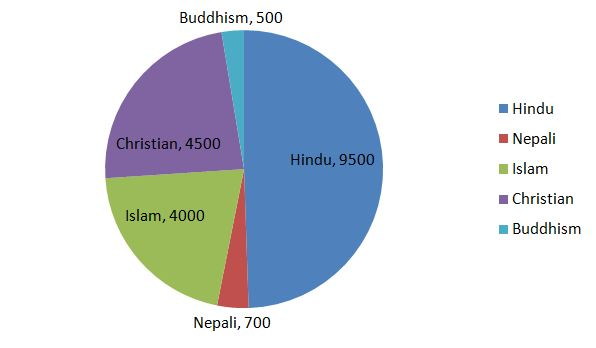Thus, we obtain the required pie chart, shown in the above figure.

3. Manohar lists his monthly expenditure as follows:

Expenditure Food Rent Personal Insurance Health Care Entertainment Education Clothing Others
Amount (in rupees) 500 1500 850 640 360 300 350 1700

Draw a pie chart for the Manohar monthly expenditure.

Solution:

Given table says the monthly expenditure of a person.

Total expenditure in a month = 500 + 1500 + 850 + 640 + 360 + 300 + 350 + 1700 = 6200

Central angle for a component = (Value of the component / Sum of the values of all components) * 360°

Calculation of central angles:

Expenditure Amount Central Angle
Food 500 (500 / 6200) * 360 = 29°
Rent 1500 (1500 / 6200) * 360 = 87°
Personal Insurance 850 (850 / 6200) * 360 = 49.35°
Health care 640 (640 / 6200) * 360 = 37.16°
Entertainment 360 (360 / 6200) * 360 = 20.9°
Education 300 (300 / 6200) * 360 = 17.41°
Clothing 350 (350 / 6200) * 360 = 20.32°
Others 1700 (1700 / 6200) * 360 = 98.7°

Steps for constructing a pie chart:

1. Draw a circle of any convenient radius.

2. Draw a horizontal radius of the circle.

3. Draw sectors starting from the horizontal radius with central angles of 29°, 87°, 49.35°, 37.16°, 20.9°, 17.41°, 20.32°, and 98.7° respectively.

4. Shade the sectors differently using different colors and label them.Thus, we obtain the required pie chart, shown in the above figure.

4. There are 2000 workers in a factory as per the list provided below:

Cadre Laborer Mechanic Fitter Plumber Electrician Supervisor Clerk
Number of Workers 368 560 300 280 500 152 120

Represent the above data by a pie chart.

Solution:

The total number of workers in a factory = 2000

Central angle for a component = (Value of the component / Sum of the values of all components) * 360°

Calculation of the central angle of each cadre is as follows:

Cadre No of Employees Central Angle
Laborer 368 (368 / 2000) * 360 = 66.24°
Mechanic 560 (560 / 2000) * 360 = 100.8°
Fitter 300 (300 / 2000) * 360 = 54°
Plumber 280 (280 / 2000) * 360 = 50.4°
Electrician 500 (500 / 2000) * 360 = 90°
Supervisor 152 (152 / 2000) * 360 = 27.36°
Clerk 120 (120 / 2000) * 360 = 21.6°

Construction for creating a pie chart

1. Draw a circle of any convenient radius.

2. Draw a horizontal radius of the circle.

3. Draw sectors starting from the horizontal radius with central angles of 66.24°, 100.8°, 54°, 50.4°, 90°, 27.36°, and 21.6° respectively.

4. Shade the sectors differently using different colors and label them.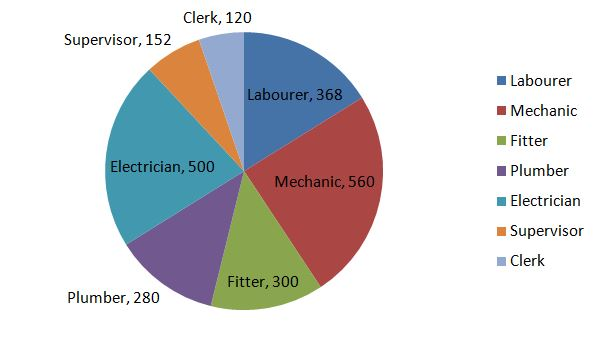Thus, we obtain the required pie chart, shown in the above figure.

5. The following table gives the number of different fruits kept in a hamper.

Type of Fruit Apple Mango Papaya Orange Pomegranate Supervisor Clerk
Number 368 560 300 280 500 152 120

Draw a pie chart to represent the above data.

Solution:

The total number of fruits in the hamper = 80

Central angle for a component = (Value of the component / Sum of the values of all components) * 360°

Calculation of central angles:

Type of Fruit Numbers Central Angle
Apple 12 (12 / 80) * 360 = 54°
Mango 20 (20 / 80) * 360 = 90°
Papaya 5 (5 / 80) * 360 = 22.5°
Orange 15 (15 / 80) * 360 = 67.5°
Pomegranate 18 (18 / 80) * 360 = 81°
Kiwi Fruit 10 (10 / 80) * 360 = 45°

Construction for creating a pie chart

• Draw a circle of any convenient radius
• Draw sectors starting from the horizontal radius with central angles of 54°, 90°, 22.5°, 67.5°, 81°, and 45° respectively.
• Shade the sectors differently using different colors and label them.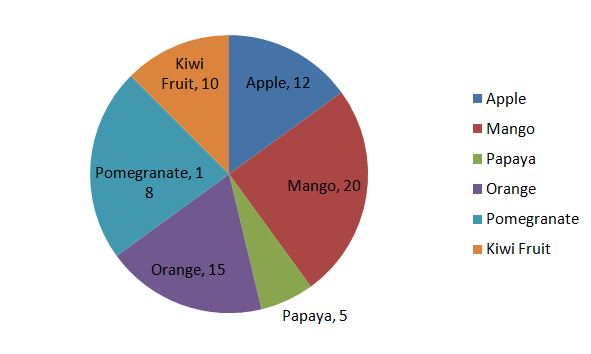Thus, we obtain the required pie chart, shown in the above figure.

6. The data given below shows the number of hours spent by working women on different activities on a working day.

Activity Office Cooking Sleep Journey Others
Hours 8 2 7 2 5

Represent the above data by a pie chart.

Solution:

Given data is about the hours spent by working women.

Total number of hours = 24

Central angle for a component = (Value of the component / Sum of the values of all components) * 360°

Calculation of central angles:

Activity Hours Central Angle
Office 8 (8 / 24) * 360 = 120°
Cooking 2 (2 / 24) * 360 = 30°
Sleep 7 (7 / 24) * 360 = 105°
Journey 2 (2 / 24) * 360 = 30°
Others 5 (5 / 24) * 360 = 75°

Construction for creating a pie chart

Steps of construction:

1. Draw a circle of any convenient radius.

2. Draw a horizontal radius of the circle.

3. Draw sectors starting from the horizontal radius with central angles of 12°, 30°, 105°, 75°, and 30° respectively.

4. Shade the sectors differently using different colors and label them.Thus, we obtain the required pie chart, shown in the above figure.

7. 500 students of a school were surveyed for their favorite activity. The information is represented in a pie chart as given below. Observe the chart and answer the questions given below.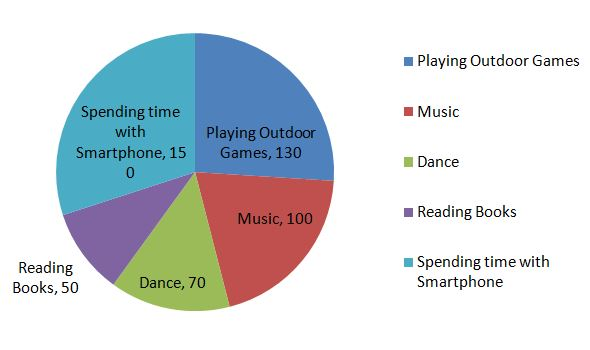(i) What is the pie chart tells about?

(ii) Which is the most favorite pastime activity of students?

(iiI) How many students like reading books?

(iv) What percentage of students like music?

(v) What percentage of students like to dance?

(vi) What percentage of students like playing outdoor games?

Solution:

From the pie chart, we can observe that,

(i) Pie chart contains the 500 school students’ favorite activity.

(ii) Spending time with the smartphone is the favorite past activity for most of the school students.

(iii) Out of 500 students, 50 students like reading books.

(iv) 100 students like music. The percentage of students who like music is (100 / 500) * 100 = 20%

(v) 70 students like dance. The percentage of students who like dance is (70 / 500) * 100 = 14%

(vi) 130 students like playing outdoor games. The percentage of students who like playing outdoor games is (130 / 500) * 100 = 26%

8. Given the pie chart shows the sales of different brands of smartphones on an e-commerce site during a festive season.From the pie chart, solve the following questions.

(i) What are the different brands mentioned in the chart?

(ii) How many smartphones are sold during the festive season?

(iii) How many Nokia smartphones are sold?

(iv) Which brand of the smartphone has the highest sales and lowest sales?

(v) What is the difference between the sales of apple and Motorola?

(vi) What is the ratio of sales between Sony and Samsung?

Solution:

The pie chart is given about the sales of different brands of smartphones in an e-commerce site during a festive season.

(i) The different brands mentioned in the chart are Motorola, Coolpad, Apple, HTC, Sony, Nokia, Lenovo, and Samsung.

(ii) A total of 10,000 smartphones are sold during the festive season in an e-commerce season.

(iii) A total of 550 Nokia brand smartphones are sold.

(iv) Lenovo has the highest sales and Coolpad has the lowest sales.

(v) The number of Apple smartphones sold is 600, Motorola smartphones are 664. So, the difference between the sales of Motorola and apple is 664 – 600 = 64.

(vi) The sales of Sony is 1500, Samsung is 2500. The ratio between the sales of sony and Samsung is 1500 : 2500 = 3 : 5.

9. The pie chart below shows the percentages of types of transportation used by 500 students to come to school.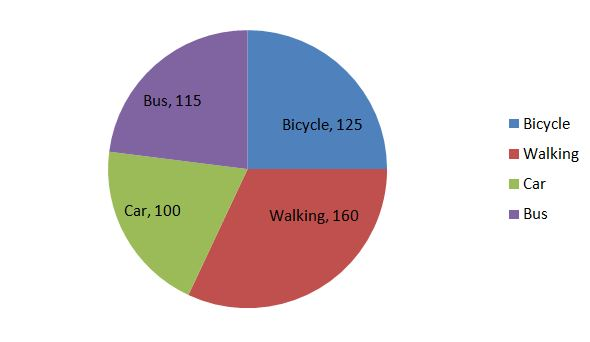(i) How many students come to school by bicycle?

(ii) How many students do not walk to school?

(iii) How many students come to school by bus?

(iv) How many students come to school by car?

(v) What is the central angle for students to come to school by walk?

(vi) What is the ratio between students come to school by car, by bus?

Solution:

(i) 125 students come to school by bicycle.

(ii) (115 + 100 + 125) = 340 students do not walk to school.

(iii) 115 students come to school by bus.

(iv) 100 students come to school by car.

(v) 160 students come to school by walk. The central angle for students come to school by walk = (160 / 500) * 360 = 0.32 * 360 = 115.2°

(vi) 100 students come to school by car, 115 students come to school by bus. The ratio is 100 : 115 = 20 : 23

10. The following are the ingredients and quantity of ingredients required to make a butter cake.

Ingredients Flour Sugar Egg Butter
Quantity 30 20 35 10

Draw a pie chart.

Solution:

Total quantity = 95

Calculation of central angles:

Ingredients Quantity Central Angle
Flour 30 (30 / 95) * 360 = 113.6°
Sugar 20 (20 / 95) * 360 = 75.7°
Egg 35 (35 / 95) * 360 = 132.6°
Butter 10 (10 / 95) * 360 = 37.8°

Construction for creating a pie chart

Steps of construction:

1. Draw a circle of any convenient radius.

2. Draw a horizontal radius of the circle.

3. Draw sectors starting from the horizontal radius with central angles of 113.6°, 75.7°, 132.6°, and 37.8° respectively.

4. Shade the sectors differently using different colors and label them.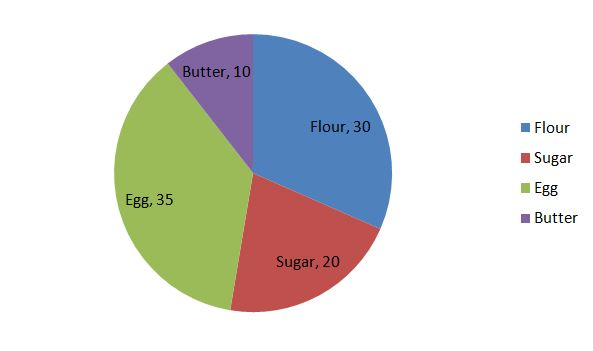Thus, we obtain the required pie chart, shown in the above figure.

11. In the pie chart representing the percentages of students having an interest in playing various kinds of games, the central angle of the sector representing students playing cricket is 152°, what is the percentage of students interested in playing cricket?

Solution:

Given the central angle of the sector = 152°

(152 / 360) * 100 = 42 %

The percentage of students interested in playing cricket is 42 %.

12. In a pie chart representing the number of students opting for different modes of transportation to reach the school. The total number of students is 1000, and the central angle of the sector representing transportation by walk is 52°, what is the number of students who opted for the mode of transportation is by walk?

Solution:

Given that,

The central angle of the sector for walk= 52°

The total number of students = 1000

Central angle for a component = (Value of the component / Sum of the values of all components) * 360°

52° = (Value of the component / 1000) * 360°

52 / 360 = Value of the component / 1000

0.1444 = Value of the component / 1000

Value of the component = 0.144.4 * 1000

Value of the component = 144.4

The number of students who opted walking to reach the school = 144

13. If 40% of the people’s favorite food is biryani then the central angle of the sector representing the people’s favorite food in a pie chart would be.

Solution:

Given that,

The percentage of the number of people favorite food is biryani = 40%

Central angle for a component = (Value of the component / Sum of the values of all components) * 360°

= (40 / 100) * 360

= 144°

The central angle of the sector whose favorite food is biryani is 144°.

14. A person’s monthly income is \$ 5200 and his monthly expenditure on food is \$ 500. What is the central angle of the sector representing food expenses in the pie chart?

Solution:

Given that,

Person monthly income = \$ 5200

Expenditure on food = \$ 500

The central angle of food = (Expenditure of food/person monthly income) * 360°

= (500 / 5200) * 360°

= 0.096 * 360°

= 34.61°

The central angle of the sector representing food expenses in the pie chart = 34.61°.

15. The following table shows the percentage of buyers of six different brands of bathing soaps.

Brand A B C D E F
Percentage of Buyers 10 15 5 40 15 15

Represent data on a pie chart.

Solution:

The given table represents the percentage of buyers of six different brands of bathing soaps.

Central angle for a component = (Value of the component / Sum of the values of all components) * 360°

Calculation of central angles:

Brand Percentage of Buyers Central Angle
A 10 (10 / 100) * 360 = 36°
B 15 (15 / 100) * 360 = 54°
C 5 (5 / 100) * 360 = 18°
D 40 (40 / 100) * 360 = 144°
E 15 (15 / 100) * 360 = 54°
F 15 (15 / 100) * 360 = 54°

Construction for creating a pie chart

Steps of construction:

1. Draw a circle of any convenient radius.

2. Draw a horizontal radius of the circle.

3. Draw sectors starting from the horizontal radius with central angles of 36°, 54°, 18°, 144°, 54°, and 54° respectively.

4. Shade the sectors differently using different colors and label them.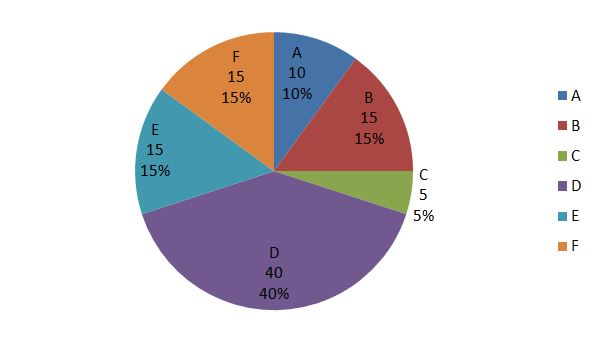Thus, we obtain the required pie chart, shown in the above figure.